# 2nd PUC Basic Maths Question Bank Chapter 19 Differential Calculus

Students can Download Basic Maths Question Bank Chapter 19 Differential Calculus Questions and Answers, Notes Pdf, 2nd PUC Basic Maths Question Bank with Answers helps you to revise the complete Karnataka State Board Syllabus and to clear all their doubts, score well in final exams.

## Karnataka 2nd PUC Basic Maths Question Bank Chapter 19 Differential Calculus

### 2nd PUC Basic Maths Differential Calculus One Mark Questions and Answers

Question 1.
Find the Marginal revenue for the demand function 3x – x2.
Total revenue. = demand function × x .
= (3x – x2)x = 3x2 – x3
Marginal revenue = $$\frac{d}{d x}$$(T.R) = 6x – 3x2.

Question 2.
A company has revenue function R = 100Q – Q2. Find the marginal revenue function (Q is the output)
M.R = $$\frac{d}{d Q}$$(T.R) = $$\frac{d}{d Q}$$(100Q – Q2) = 100 – 2Q

Question 3.
If the (Total Revenue) T.R is 3q2 – 4q + 3 find the Average Revenue.
Average Revenue = $$\frac{T \cdot R}{Q}=\frac{3 q^{2}-4 q+3}{Q}=3 Q-4+\frac{3}{Q}$$Question 4.
If S = 4t3 + 9t2 + 5t, find the initial velocity where S is the distance in meters travelled by a particle in time t secs.
Velocity = $$\frac{d s}{d t}=\frac{d}{d t}$$ (4t3 + 9t2+ 5Q
= 12t2 + 18t + 5
initial velocity = Vt=0 = 5 m/sec.

Question 5.
A Particle moves in a striaght line. Distance S is given by S = 2t2 + 5t – 3. Find the velocity when t = 4 secs
Vt = 4 = $$\frac{d s}{d t}=\frac{d}{d t}$$ (2t2 + 5t – 3) = 4t + 5 = 4. 4 + 5
= 16 + 5 = 21 m/sec.

Question 6.
The demand function of a firm is given by P = 50 – 2x where P is the given price for x units determine the marginal revenue.
T.R = Price × x = (50 – 2x) x = 50x – 2x2
M.R= $$\frac{d}{d x}$$(T.R) = $$\frac{d}{d x}$$(50x – 2x2) = 50 – 4x.

Question 7.
The Total cost function of a firm is given by C(x) = 2x3 – x2 + 5x. Find the average and Marginal cost functions.
Average cost = $$\frac{\text { Total cost }}{x}=\frac{2 x^{3}-x^{2}+5 x}{x}$$
= 2x2 – x + 5
Marginal cost = $$\frac{d}{d x}$$(T.C) = $$\frac{d}{d x}$$(2x3 – x2 + 5x) = 6x2 – 2x + 5

Question 8.
The Total revenue and Total cost functions are given by R(x) = 30x – x2 and C(x) = 20 + 6x. Find x if M.R = MC (x is the output).
M.R = $$\frac{d}{d x}$$(T.R) = $$\frac{d}{d x}$$(30x – x2) = 30 – 2x
M.C = $$\frac{d}{d x}$$(T.C) = $$\frac{d}{d x}$$(20 + 6x) = 6
MR = MC
30 – 2x = 6 ⇒ 30 – 6 = 2x ⇒ x = 12.Question 9.
Divide the numbers 1238 in to 2 parts such that their product is maximum.
Let the 2 parts of the number = x & 1238 – x
Let y = x(1238 – x) = 1238x – x2
$$\frac{dy}{d x}$$ = 1238 – 2x = 0 ⇒ x = 619
$$\frac{d^{2} y}{d x^{2}}$$ = -2 < 0 the function is maximum dx
∴ The two parts are 619 dnd 619.

Question 10.
If S = 4t2 + 7t + 9. Find the acceleration at t = 3sec
V = $$\frac{d s}{d t}=\frac{d}{d t}$$(4t2 + 7t + 9)
V = 8t + 7 dv
accn = $$\frac{d v}{d t}$$ = 8 units/sec. dt

Question 11.
If S = $$\sqrt{1-t}$$ where S is the displacement P.T Velocity varies inversely as displacement.
S = $$\sqrt{1-t}$$
diff w.r.t t we get velocityQuestion 12.
Find a and b such that y = ab is maximum given a + b = 20.
a + b = 20 ⇒ b = 20 – a
y = ab
y = a(20 – a)
y = 20a – a2 diff w.r.t a
$$\frac{d y}{d a}$$ = 20 – 2a, $$\frac{d y}{d a}$$ = 0 ⇒ 20 – 2a = 0 ⇒ a = 10
$$\frac{d^{2} y}{d x^{2}}$$ = -2 < 0 ∴ y has maximum value.
∴a = 10 & b = 10Question 13.
If S = 4t2 – 2t + 3 find (i) velcocity (ii) acceleration at t = 2 sec.
Velocity = $$\frac{d s}{d t}$$ = 8t – 2
Vr=2sec = 8.2 – 2 = 16 – 2 = 14 unit/sec
acceleration = $$\frac{d v}{d t}$$ = 8 units2/sec.

Question 14.
A Cylindrical tank is 8 mts in diameter, Water is flowing into it at the rate of 36 cubic mt3/sec. Find the rate at which the water level is rising.
V = πr2h r = constent, Give r = 4 mts $$\frac{d v}{d t}$$ = 36cc/sec
$$\frac{d v}{d t}$$ = πr2h . $$\frac{d h}{d t}$$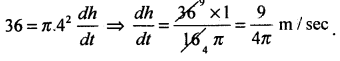Question 15.
A square plate expands uniformly the side is increasing at the rate of 5cm/sec. What is the rate of increase in area when the side is 10cm?
A = x2 Gìven $$\frac{d x}{d t}$$ = 5cm/sec. x = 10cm.
$$\frac{d A}{d t}$$ = 2x . $$\frac{d x}{d t}$$ = 2 × 10 × 5 = 100cm2/sec

Question 16.
The radius of a circular blot of ink is increasing at the rate of 3cm/mm. Find the rate of increase of its area when its radius is 2cm.
A = πr2 Given $$\frac{d x}{d t}$$ = 3cm/min, r = 2cm
$$\frac{d A}{d t}$$ = π.2r. $$\frac{d r}{d t}$$
= π. 2. 2.3 = 12π sq cm/min.Question 17.
If C is the total cost for producing x – units of a product and the average cost function is given by 0.003x2 – 0.04x + 6 + $$\frac{3000}{x}$$ Find the marginal cost function.
Given Ave. cost = 0.003x2 – 0.04x + 6 + $$\frac{3000}{x}$$
Total cost = (Ave – Cost) × x
= 0.003x3 – 0.04x2 + 6x + 3000
and Marginal cost = $$\frac{d}{d x}$$(T.C)
= 0.009x2 – 0.08x + 6.

Question 18.
If S = t3 – 6t2 + 9t + 8 where S is the distance travelled by a particle in t secs then find
(i) Initial velocity and (ii) when the body will be at rest momentarily.
Given S = t3 6t2 + 9t + 8
V = $$\frac{d S}{d t}$$ = 3t2 – 12t + 9
Initial velocity = 9 units / sec (when t = 0)
Body will be at rest when velocity = 0
3(t2 – 4t + 3) = 0 ⇒ t2 – 4t + 3 = 0
(t – 1)(t – 3) = 0 ⇒ t = 1 sec or 3 sec.### 2nd PUC Basic Maths Differential Calculus Three or Four Marks Questions and Answers

Question 1.
If S = at3 + bt, find a and b given t = 13 secs, velocity is 0 and acceleration 14 units.
Given S = at3 + bt
V = $$\frac{d S}{d t}$$ = 3at2 + b
V = 0 at t = 3 secss
3a.2 + b = 0 ⇒ 27a + b = 0
acceleration = $$\frac{d v}{d t}$$ = 6at
Given 14 = 6a.3
14 = 18a ⇒ a = $$\frac{14}{18}=\frac{7}{9}$$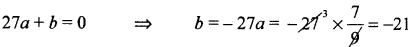a = $$\frac { 7 }{ 9 }$$ b = -21.

Question 2.
A particle moves at a distance S = 6t2 – t2 + 5 then find (i) The velocity and acceleration after 3 sec (ii) The time at which it is rest.
(i) V = $$\frac{d s}{d t}$$ = 12t – 3t2
Vr=3sec = 12.3 – 3.32 = 36 – 27 = 9mt/sec
acceleration = $$\frac{d v}{d t}$$ = 12 – 6t
At t = sec3 = 12 – 6.3 = 12 – 18 = -6mt/sec2

(ii) At rest V = 0
∴ $$\frac{d s}{d t}$$ = 0 ⇒ 12t – 3t2 = 0
3t(4 – t)0 ⇒ t = 0 or t = 4sec
∴ the particle is at rest at 0 sec or at 4 sec.Question 3.
Find the value of X at which the function, y = 2x3 – 21x2 + 36x – 20 is maximum.
y = f(x) = 2x3 – 21x2 + 36x – 20
f1(x) = 6x2 – 42x + 36
f11(x) = 12x – 42
for a function to be maxm f1(x) = 0
⇒ 6(x2 – 7x + 6) = 0
⇒ (x – 1)(x – 6) = 0 x = 1 or 6
Put x = 1 in f’11(x)
f’11(1) = 12 – 42 = – 30 < 0 ∴f(x) has maxm at x = 1 at x = 1
put x = 6 in f11(x)
f11(6) = 72 – 42 = -30 > 0 ∴ f(x) has minm at x = 6.

Question 4.
The cost C = 5 + $$\frac{48}{x}$$ + 3x2 where x is the number of articles manufactured. Find the minimum value of C.
C(x) = 5 + $$\frac{48}{x}$$ + 3x2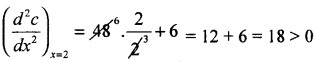∴ Cost has minimum at x = 2.Question 5.
If S = at2 + 2bt + C, where S is the displacement at time t and a, b, c are constants S.T. The acceleration is inversely proportional to S3.
Given S = at2 + 2bt + C
2S $$\frac{d s}{d t}$$ = 2at + 2b∴acceleration is inversely proportion to s1

Question 6.
The cost function of a firm is given as C(x) 300x – 10x2 + $$\frac{x^{3}}{3}$$ where C stands for total cost and x for output. Calculate the output when AC = MC.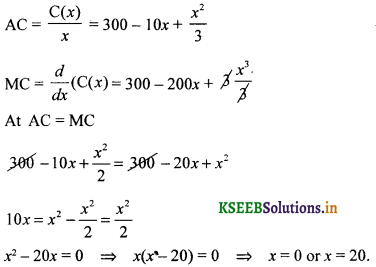Question 7.
The Volume of a sphere is increasing at the rate of 6 C.C/sec. Find the rate of increase of the radius when the radius is 4 cms.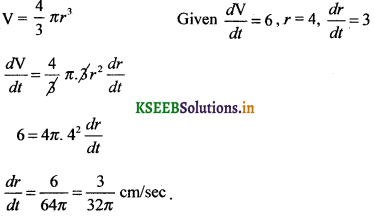Question 8.
Find the total revenue, Margin! revenue and A.R when the demand function is given as Q = 30 – 4P + P2 where P is the price and Q is the Quantity demanded also calculate the marginal revenue when P = 3.
T.R = PQ
=P(30 – 4P + P2)
= 30P – 4P2 + P3
M.R = (T.R)
M.R = 30 – 8P + 3P2
At P = 3, M.R = 30 – 24 + 27 = 33
A.R = $$\frac{\mathrm{T} \cdot \mathrm{R}}{\mathrm{Q}}$$ = 3Q – 4p – p2Question 9.
A manufacturer has a total cost C 100 + 32√x . He would like to know at what level of output his MC will be Rs 2.
T.C = 100 + 32√x
M.C = 32$$\frac{1}{2 \sqrt{x}}$$
2 = $$\frac{32}{2 \sqrt{x}}$$ ⇒ √x = 8 ⇒ x = 64

Question 10.
If the total cost function is C = 8x3 – 12x2 + 20x where x is th. level of output. Find X at which the ave cost is minimised. Find theT.C for this output.
T.C = C = 8x3 – 12×2 + 20x
A.C = $$\frac{T . C}{x}$$ = 8x2 – 12×2 + 20x
Ave cost is minimised ∴ $$\frac{d}{d x}$$ (A.C) = 0
⇒ 16x – 12 = 0 ⇒ x= $$\frac{3}{4}$$Question 11.
The sides of an Equilateral triangle are increasing at the rate of 2cm/sec. How fast its area increasing when the sides are 10 cm.
A = $$\frac{\sqrt{3}}{4}$$x2 Given $$\frac{d x}{d t}$$ = 2 , x = 10cm, $$\frac{d a}{d t}$$ = ?
$$\frac{d A}{d t}=\frac{\sqrt{3}}{4} \cdot 2 x \cdot \frac{d x}{d t}$$Question 12.
The heights of a cone is 60 cm and it is constent. The radius of the base is increasing at the rate of 0.50cmlsec. Find the rate of increase of Volume of the cone when the radius is 10cm.
V = $$\frac{1}{3}$$πr2h Given h = 60 cm constent
$$\frac{d v}{d t}$$ = $$\frac{1}{3}$$ πh . 2r $$\frac{d r}{d t}$$ r = 10 cm
= $$\frac{2}{3}$$π × 60 × 10 × 0.5 $$\frac{d r}{d t}$$ = 0.50cm/sec
= 200 cm2/sec.Question 13.
The Edge of a variable cube is increasing at the rate of 6cm/min. How fast is the volume and its surface increasing when the edge is 10cm long?
V = x3 Given $$\frac{d x}{d t}$$ =6 cm/rn in
$$\frac{d v}{d t}$$ = 3x2 $$\frac{d x}{d t}$$ x = 10cm, $$\frac{d x}{d t}$$ = ?, $$\frac{d A}{d t}$$ = ?
= 3.102.6
= 1800 cm3/min
A = 6x2.
$$\frac{d A}{d t}$$ = 12x . $$\frac{d x}{d t}$$ = 12.10.6 = 720cm2/min.

Question 14.
A ladder of 15ft leans against a vertical wall. If the top slides downwards at the rate of 3 ft/sec. Find how fast the lower end is moving when ¡t is 12 ft from the waIl. , 15
x2 + y2 = 152 ⇒ y2 = 152 – 122 = 81
y = 9### 2nd PUC Basic Maths Differential Calculus Five Marks Questions and Answers

Question 1.
A boy 150 cms tall walks directly away from a light source which is 600cms above the ground at 6 kmslhour. Find the rate at which (i) the tip of shadow is moving (iii) his shadow 600 m lengthens.
Let AB be the light source
CD be man
BD be the distance by which a man walks away from a light source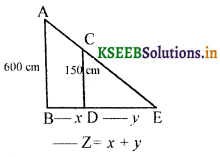Given $$\frac{d y}{d t}$$ = 6, $$\frac{d x}{d t}$$ = ?, $$\frac{d z}{d t}$$ = ?
From the 2 similar triangles we get
$$\frac{\mathrm{AB}}{\mathrm{CD}}=\frac{\mathrm{BE}}{\mathrm{DE}}$$
$$\frac{600}{150}=\frac{x+y}{\mathrm{X}}$$ ⇒ 600x = 150x + 150y
450x = 150y
∴ $$3 \frac{d x}{d t}=\frac{d y}{d t}=6$$
∴ $$\frac{d x}{d t}=2$$
The tip of his shadow moving 2 kms/ hour and tip of the shawdow lengthens is,
$$\frac{d Z}{d t}=\frac{d x}{d t}+\frac{d y}{d t}$$ = 2 + 6 + 8 km/hr.Question 2.
A point source of light is long 25ft directly above a straight horizontal path on which a boy of 6ft high is working. flow fast is the boy’s shadow lengthening and how fast the tip of the shadow is moving when he is walking away from the light at the rate of 100 ft/min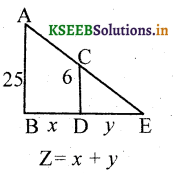Given $$\frac{d x}{d t}$$ = 100 ft/mm, $$\frac{d y}{d t}$$ = ?, $$\frac{d Z}{d t}$$ = ?
From 2 similar traingle we have
$$\frac{25}{6}=\frac{x+y}{y}$$
25y = 6x + 6y
19y = 6x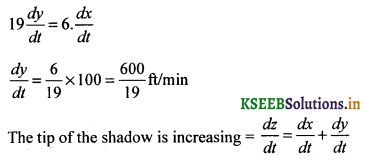Question 3.
The demand function of a company is given by P(q) = 800 – 0.4q and the total cost function is given by C(q) = 80q + 8000, where q is the level of output and Pis the price per unit. Find the level of output and the price charged which maximises the profit.
Given Demand function = P = 800 – 0.4q
Total cost C = 80q + 8000
Marginal cost = $$\frac{d}{d q}$$(T.C) = 80
Total revenue = P × Q = (800 – 0.4q)q
= 800q – 0.4q2
Marginal revenue = $$\frac{d}{d Q}$$(TR) = 800 – 0.8q
Profit is Maximum M.R M.C
800 – 0.8q = 80
800 – 800.8q ⇒ q = $$\frac{720}{0.8}$$ = 900 units
Total Profit = T.R — T.C
= 800q – 0.4q2 – 80q – 8000
= 720q – 0.4q2 – 8000
= 720 × 900 – (0.4) (900)2 – 8000
∴ Total Profit = 3,16,000.Question 4.
The total cost os production of a firm is given the function C = 0.7x + 18 find
(a) The total cost for an output of 10 units
(b) The average cost for an output of 9 units
(c) The marginal cost for an output of 6 units
(d) The profit function, if the Quantity is sold at Rs 5 per unit.
Given C = 0.7x + 18
(a) Total cost when x = 10 is C = 0.7 × 10 + 18 = 25

(b) Ave cost when x = 9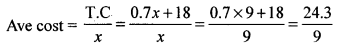(c) Marginal cost when x = 6
M.C = $$\frac{d}{d x}$$(T.C) = 0.7

(d) Profit function when selling price is Rs. 5/unit
T.R = 5x
T.C = 0.7x + 18
Profit P(x) = T.R – T.C
= 5x – 0.7x – 18
= 4.3x – 18.

Question 5.
Find the M.R when the Quantity sold is 5 units for the demand function P = 300 – Q2. If F.C is Rs. 50 and V.C is Rs 3. At what Quantity will profit be maximum.
T.R = Price × Quantity = (300 – Q2)Q = 300 Q – Q3
T.C = F.C + V.C = 50 + 3Q
M.R = $$\frac{d}{d Q}$$(T.R)300 – 3Q2.
= 300 – 3.52 (Q = 5)
= 225
Profit TR – TC = 300Q – Q3 – 3Q – 50
= 297Q – Q3 – 50
Profit is maximum if $$\frac{dp}{d Q}$$ = 0
= 297 – 3Q2 = 0 ⇒ Q = 99
∴$$\frac{d^{2} \mathrm{P}}{d \mathrm{Q}^{2}}$$ = -60 < 0
the profit is maxm at Q = 99.Question 6.
The volume of a spherical ball is increasing at the rate of 4π c.c/sec. Find the rate of increase of radius and that of surface area when the radius = 6cm.
V = $$\frac{4}{3}$$πr3 Given $$\frac{d V}{d t}$$ = 4π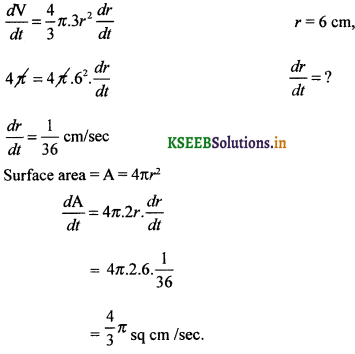Question 7.
Find the maximum and minimum values of the function f(x) = x3 – 9x2 + 15x – 3.
Given f(x) = x3 – 9x2 + 15x – 3 ______ (1)
f1(x) = 3x2 – 18x + 15 _____ (2)
f11(x) = 6x – 18 ______ (3)
For a function to be maxtm or mmm we have f1(x) = 0
3x2 – 18x + 15 = 0 + 3
x2 – 6x + 5 = 0
(x – 1)(x – 5) = 0 x = 1 or 5.

Case I: Put x = 1 in eqn (3)
f11(x) = 6 – 18 = – 12 < 0 the function is
Maxm at x = 1 & Maxm value is
ymax = 13 – 9 . 12 + 15.1 – 3 = 4

Case 2: Put z = 5 in eqn (3)
f11(x) = 30 – 18 12 > 0 the function is
Minm at x = 5 & Minm value is
ymin = f(5) = 53 – 9.52 + 15.5 – 3
ymin = -28.Question 8.
Divide 84 in to two parts such that the product of one part and the square of the other parts is maximum.
$$\frac{d y}{d x}$$ =168x – 3x2
$$\frac{d^{2} y}{d x^{2}}$$ = l68 – 6x
for a function to be maxm = $$\frac{d y}{d x}$$ = 0
Put x = 56 in $$\frac{d^{2} y}{d x^{2}}$$